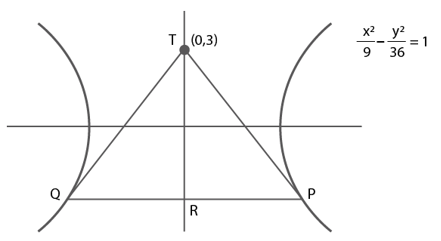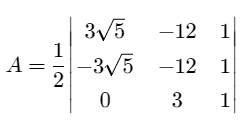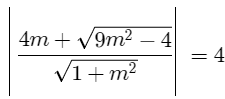# JEE Hyperbola Previous Year Questions With Solutions

JEE Previous Year Questions With Solutions on Hyperbola are provided here in PDF format, which can be downloaded for free. In order to help students, understand the concepts clearly, we are offering a comprehensive set of previous year questions with solutions. These solutions will also help students boost their preparation for JEE Main and Advanced. Students will further get to learn concepts like: equation of hyperbola under different conditions, normal and tangents of hyperbola, Vertex, focii, eccentricity, axes, applications of hyperbola and many more. Previous year chapter-wise solutions will help students understand the concepts in a better way and pattern of questions. Students can download the pdf by clicking on the given link below and start practice.

## JEE Main Past Year Questions With Solutions on Hyperbola

Question 1: The locus of a point P(α, β) moving under the condition that the line y = αx + β is a tangent to the hyperbola x2/a2 – y2/b2 = 1 is

(a) an ellipse

(b) a circle

(c) a hyperbola

(d) a parabola

Solution:

Tangent to the hyperbola x2/a2 – y2/b2 = 1 is y = mx ± √(a2m2 – b2)

Given that y = αx + β is the tangent of hyperbola.

m = α and a2m2 – b2 = β2

Therefore, a2 α 2 – b2 = β2

Locus is a2 x 2 – y2 = b2 , which is parabola.

Question 2: If a hyperbola passes through the point P(10, 16) and it has vertices at (±6, 0), then the equation of the normal at P is:

(a) 3x + 4y = 94

(b) x + 2𝑦 = 42

(c) 2x + 5y = 100

(d) x + 3y = 58

Solution:

Vertex of hyperbola: (±a, 0) = (± 6, 0) => a = 6

We know, equation of hyperbola, x2/a2 – y2/b2 = 1

=> x2/36 – y2/b2 = 1

Point P(10,16) lies on parabola, so

100/36 – 256/b2 = 1

=> 64/36 = 256/b2

=>b2 = 144

Equation of hyperbola becomes, x2/36 – y2/144 = 1 and

Equation of normal : a2x/x1 + b2y/y1 = a2 + b2

=> 36x/10 + 144y/16 = 180

=> x/50 + y/20 = 1

Or 2x + 5y = 100

Question 3: The eccentricity of the hyperbola whose latus rectum is 8 and length of the conjugate axis is equal to half the distance between the foci, is

(a) √3 (b) 4/3 (c) 2/√3 (d) 4/√3

Solution:

We know, conjugate axis of hyperbola = 2b and

Latus rectum = 2b2/a

Given: The eccentricity of the hyperbola whose latus rectum is 8 and length of the conjugate axis is equal to half the distance between the foci.

=> 2b = 1/2(2ae) and 2b2/a = 8

=>2/a(ae/2)2 = 8

=> ae2 = 16 …(i)

Also, we know, b2 = a2 (e2 – 1)

From equation, 2b2/a = 8 =>b2 = 4a

So, a2 (e2 – 1) = 4a

=>ae2 – a = 4

Using (i)

=>16 – a = 4

Or a = 12

Again, (i)=> 12 e2 = 16

=> e = 2/√3

Question 4: An ellipse passes through the foci of the hyperbola, 9x2 −4y2 =36 and its major and minor axes lie along the transverse and conjugate axes of the hyperbola respectively. If the product of eccentricities of the two conics is 1/2. Find the equation of ellipse.

Solution:

Equation of hyperbola is 9x2 −4y2 =36 or x2/4 − y2/9 = 1

(Here a < b)

Focus = (0, ± be)

Eccentricity = e = √(1+4/9) = √13/3

So, Foci of hyperbola: (0, ±√13)

Standard equation of the ellipse, x2/a2 + y2/b2 = 1 …(i)

Eccentricity = e’ = √(1-a2/b2) …(ii)

ee’ = 1/2 (given)

Using eccentricity value of hyperbola, e’ = 1/2 x 3/√13 = 3/2√13

(ii) => e’2 = (1-a2/b2)

9/52 = (1-a2/b2)

Find the value of b2 form (i) using focii 13/b2 = 1 => b2 = 13

=> 9/52 = (1-a2/13)

=> 9/4 = 13 – a2

=> a2 = 43/4

Now equation of ellipse is 4x2/43 + y2/13 = 1

Question 5: If e1 and e2 are the eccentricities of the ellipse, x2/18 + y2/4 = 1 and the hyperbola, x2/9 – y2/4 = 1 respectively and (e1, e2) is a point on the ellipse, 15x2 + 3y2 = k. Then k is equal to

(a) 14 (b) 15 (c) 17 (d) 16

Solution:

e1 and e2 are the eccentricities of the ellipse, x2/18 + y2/4 = 1 and the hyperbola, x2/9 – y2/4 = 1 respectively

$e_1 = \sqrt{1-\frac{4}{18}} = \frac{\sqrt{7}}{3}\\$ $e_2 = \sqrt{1+\frac{4}{9}} = \frac{\sqrt{13}}{3}$

As (e1 , e2) lies on the ellipse 15x2 + 3y2 = k

Because, 15e12 + 3e22 = k

=> 15 x 7/9 + 3 x 13/9 = k

=> k = 16

Question 6: A tangent to the hyperbola x2/4 – y2/2 = 1 meets x -axis at P and y -axis at Q. Lines PR and QR are drawn such that OPRQ is a rectangle (where O is the origin). Then R lies on:

(a) 2/x2 – 4/y2 = 1

(b) 4/x2 – 2/y2 = 1

(c) 4/x2 + 2/y2 = 1

(d) 2/x2 + 4/y2 = 1

Solution:

Equation of tangent at point (a sec θ, b tanθ) is x/a secθ – y/b tanθ = 1

Equation of tangent of hyperbola x2/4 – y2/2 = 1 is (x secθ)/2 – (y tanθ)/√2 = 1 at any parametric point Q.

The coordinate of P and Q are (a cosθ, 0) and (0, -b cotθ) respectively.

OPRQ is a rectangle, Let the coordinates of point R be (h, k).

then h = a cos θ and k = -b cotθ

Therefore, secθ = a/h and tanθ = -b/k

=> a2/h2 – b2/k2 = 1

So, the required locus is 4/x2 – 2/y2 = 1

Question 7: If the eccentricity of the hyperbola $\frac{x^2}{cos^2 \theta} – \frac{y^2}{sin^2 \theta} = 1$ is more than 2 when θ Є (0, π/2) then values of length of latus rectum lies in the interval

(a) (3, ∞) (b) (1, 3/2) (c) (2, 3) (d) (-3, -2)

Solution:

We know, for hyperbola, e2 = 1 + b2/a2

= 1 + tan2 θ = sec2 θ

If e > 2 => sec θ > 2

=> θ Є (π/3, π/2)

Now, length of latus rectum of hyperbola = 2b2/a = 2 tan θ sin θ

= 2 > √3 > √3/2 > 3

Therefore, the values of length of latus rectum lies in the interval (3, ∞).

Question 8: Let the eccentricity of the hyperbola x2/a2 – y2/b2 = 1 be the reciprocal to that of the ellipse x2 + 4y2 = 4. If the hyperbola passes through a focus of the ellipse, then

(a) the equation of the hyperbola is x2/3 – y2/2 = 1

(b) the eccentricity of the hyperbola is √(5/3)

(c) a focus of the hyperbola is (2, 0)

(d) the equation of the hyperbola is x2 – 3y2 = 3

Solution:

The eccentricity of ellipse, e = √(1-b2/a2)

Given equation of ellipse x2 + 4y2 = 4 can be rewritten as x2/4 + y2/1 = 1.

eccentricity = √(1-1/4) = √3/2

Given: The eccentricity of the hyperbola x2/a2 – y2/b2 = 1 be the reciprocal to that of the ellipse x2 + 4y2 = 4.

=> eccentricity of hyperbola = 2/√3

Now,

=> √(1+b2/a2) = 2/√3

=> (1+b2/a2) = (2/√3)2

=> b/a = 1/√3

Focus of ellipse = (±ae, 0) = (±√3, 0)

Hyperbola passes through Focus, so 3/a2 = 1 => a = √3

And b/a = 1/√3 = > b/√3 = 1/√3 => b = 1

The equation of hyperbola is x2/3 – y2/1 = 1

Or x2 – 3y2 = 3

Focus of hyperbola = (±ae, 0) = (±2, 0)

Question 9: Tangents are drawn to the hyperbola 4x2 – y2 = 36 at the points P and Q. If these tangents intersect at the point T(0, 3) then find the area of △PTQ. [Area in sq. units]

Solution:

Equation of hyperbola can be written as, 4x2/36 – y2/36 = 1

or x2/9 – y2/36 = 1

Let the equation of tangent at any point P(x1, y1) is xx1/a2 – yy1/b2 = 1

As tangent is passing through the point (0, 3) (Given)

So, 0/a2 – 3y/36 = 1

=> y = -12 and

Again, 4x2 – y2 = 36

Using value of y, we have x = ±3√5So, the coordinates of P and Q are (3√5, -12) and (-3√5, -12) respectively.

Now, area od triangle TPQ,= 1/2|3√5(-12 – 3) + 12 (-3√5 – 0) + 1(-9√5 – 0)|

= 1/2|-45√5 – 36√5 – 9√5|

= 1/2|90√5|

= 45√5

Question 10: The circle x2 + y2 = 8x and hyperbola x2/9 – y2/4 = 1 intersect at the points A and B. Find the equation of a common tangent with positive slope to the circle as well as to the hyperbola.

Solution:

The equation of circle x2 + y2 = 8x can be rewritten as (x – 4)2 + y2 = 16

Tangent to hyperbola is y = mx + √(9m2-4), m> 0.

Distance from center to the tangent is:on solving above equation, we get

m = 2/√5

=> y = 2x/√5 + 4/√5

Or 2x – √5 y + 4 = 0

Therefore, 2x – √5 y + 4 = 0 is equation of a common tangent with positive slope to the circle as well as to the hyperbola.

Solve More Related Questions:

Conic Sections Previous Year Questions With Solutions

Parabola Previous Year Questions With Solutions

Ellipse Previous Year Questions With Solutions

Circle Previous Year Questions With Solutions